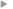## A methodology to obtain model-error covariances due to the discretization scheme from the parametric Kalman filter perspective

#### Pannekoucke, Olivier ; Ménard, Richard ; El Aabaribaoune, Mohammad ; Plu, Matthieu

Année de publication
2021
Résumé
<p align=justify>This contribution addresses the characterization of the model-error covariance matrix from the new theoretical perspective provided by the parametric Kalman filter method which approximates the covariance dynamics from the parametric evolution of a covariance model. The classical approach to obtain the modified equation of a dynamics is revisited to formulate a parametric modelling of the model-error covariance matrix which applies when the numerical model is dissipative compared with the true dynamics. As an illustration, the particular case of the advection equation is considered as a simple test bed. After the theoretical derivation of the predictability-error covariance matrices of both the nature and the numerical model, a numerical simulation is proposed which illustrates the properties of the resulting model-error covariance matrix.</p>
Texte intégralAccès à la notice sur le site du portail documentaire de Météo-FranceListe complète des notices publiques